Courses

# Organic Chemistry MCQ

## 21 Questions MCQ Test Mock Test Series of IIT JAM Biotechnology | Organic Chemistry MCQ

Description
This mock test of Organic Chemistry MCQ for IIT JAM helps you for every IIT JAM entrance exam. This contains 21 Multiple Choice Questions for IIT JAM Organic Chemistry MCQ (mcq) to study with solutions a complete question bank. The solved questions answers in this Organic Chemistry MCQ quiz give you a good mix of easy questions and tough questions. IIT JAM students definitely take this Organic Chemistry MCQ exercise for a better result in the exam. You can find other Organic Chemistry MCQ extra questions, long questions & short questions for IIT JAM on EduRev as well by searching above.
QUESTION: 1

### Among the following molecule. The most acidic molecule is: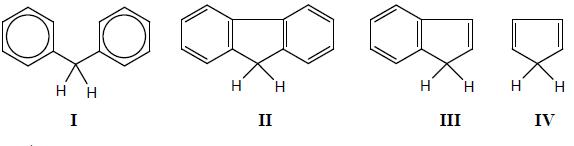Solution: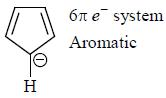Due to annelation effect compound II and IIl are less acidic.

QUESTION: 2

### The correct order of increasing reactivity towards nucleophilic addition for the following carbonyl derivative.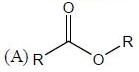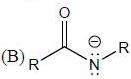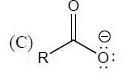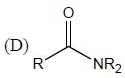Solution:

Reactivity towards nucleophile means electrophilicity. Electrophilic character at Carbonyl carbon depends upon the neighbouring group. Electron releasing group decrease electrophilicity and electron withdrawing group increase electrophilicity. Negative charge containing atom are better donar as compared to lone pair containing atom. Less electronegative atom (N) is better donar as compared to more electronegative atom (O).
So electrophilic order.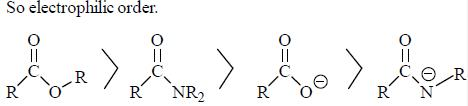QUESTION: 3

###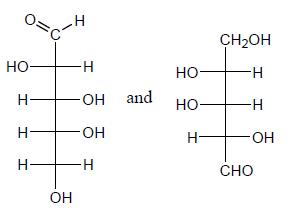The above pair is the case of

Solution: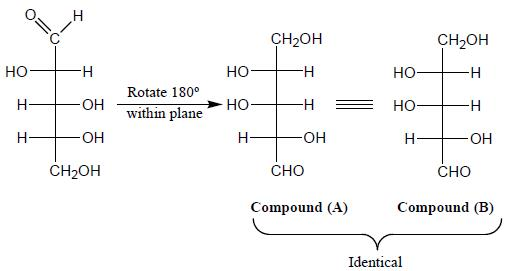QUESTION: 4

The product (P) is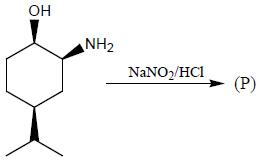Solution: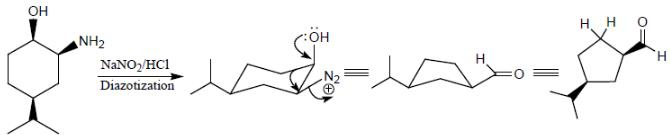QUESTION: 5

In 1H NMR spectrum of ethylbenzene, the benzylic proton appear as a

Solution: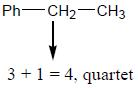QUESTION: 6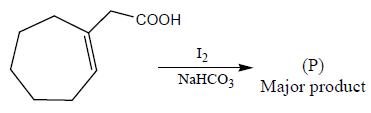Solution:QUESTION: 7

The more stable conformation of the following compound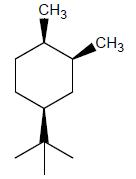Solution:

In the given compound, all substituent in cis position (above the plane). So, all group must be in up side.

QUESTION: 8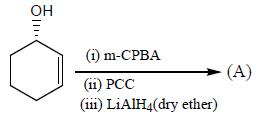What is the major product ?

Solution: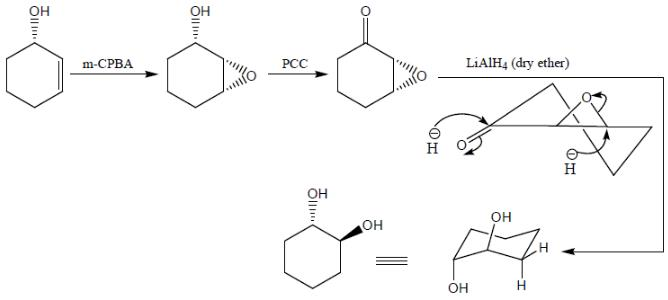QUESTION: 9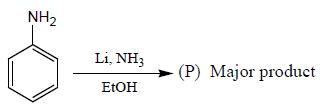Solution: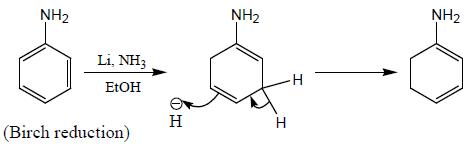QUESTION: 10

In the 300 MHz 1H NMR spectra, an organic compound exhibit doublet. Two lines of the doublet are at δ 2.35 and δ 2.38 ppm. The coupling constant is

Solution: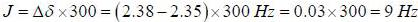*Multiple options can be correct
QUESTION: 11

Among the carbocations given below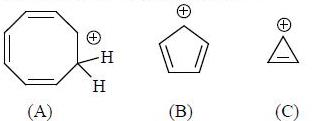Select the collect option about above compounds

Solution: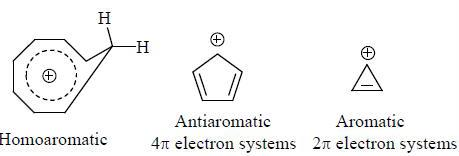*Multiple options can be correct
QUESTION: 12

The possible product’s (P) formed in the following reaction mechanism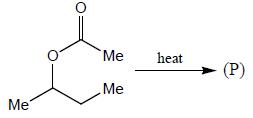Solution: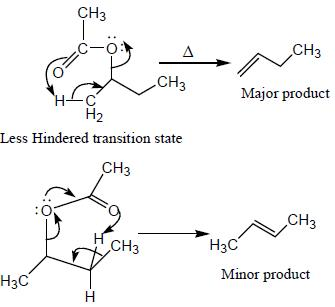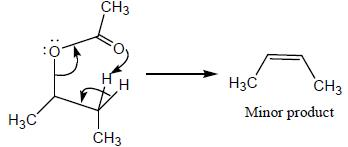QUESTION: 13

Correct decreasing order of relative rates of SN2 reactions of alkyl chlorides with iodide ion.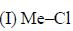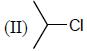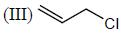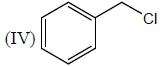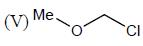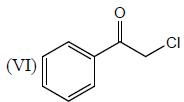Solution: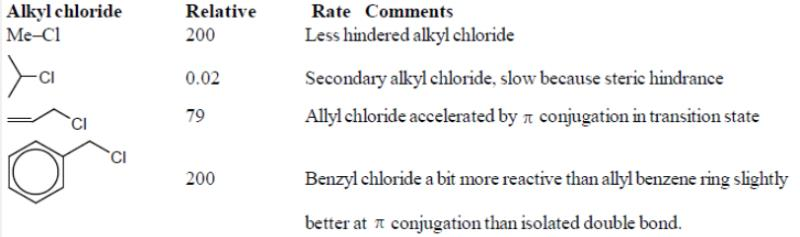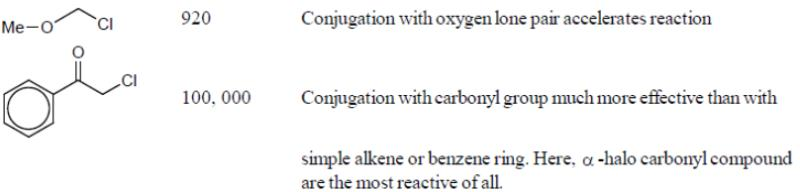*Multiple options can be correct
QUESTION: 14

Among the following which compound is non-resolvable?

Solution: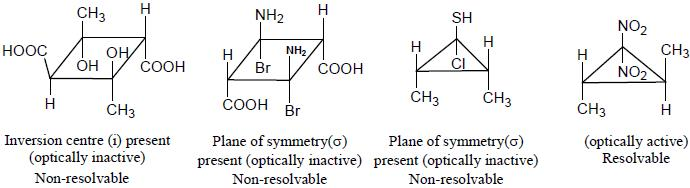*Multiple options can be correct
QUESTION: 15

Correct statements in the following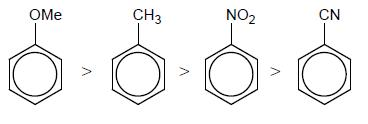Solution:

(a) Migratory apptitucle order for Pinancol-Pinacolone. rearrangement is H > Ph > R
(b) Migratoiy apptitude order for Baeyer Villiger oxidation is Ph > H > R
(c) In Backmann rearrangement, migratoiy group must be anti to hydroyl group
(d) In Pinacole Pinacolone rearrangement. the con ect order of migratoiy aptitude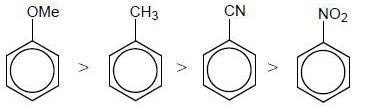*Answer can only contain numeric values
QUESTION: 16

x = number of (+M) group attached with phenyl ring, so the value of x is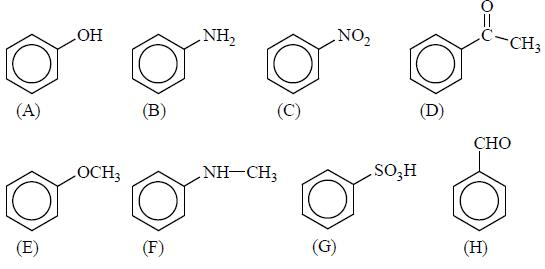Solution:

Lone pair containing atom with conjugation show (+M) effect.
A, B, E, F (+ M) effect
C, D, G, H (- M) effect

*Answer can only contain numeric values
QUESTION: 17

How many groups are O/P directive in follwing groups
(1) - NH2
(2) - CHO
(3) - N = O
(4) - COOH
(5) -OMe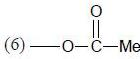(7) - CN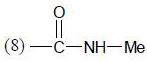(9) - N = NH2
(10) -SO3H

Solution: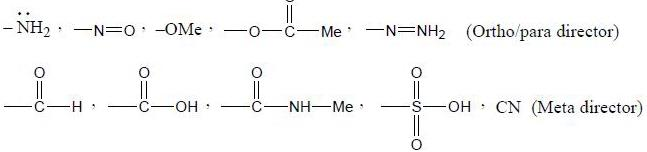*Answer can only contain numeric values
QUESTION: 18

How many carbocation can rearrange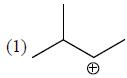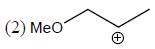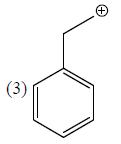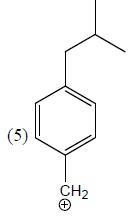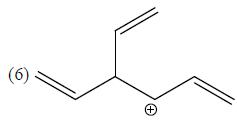Solution: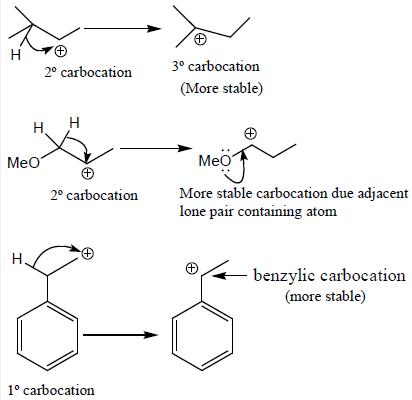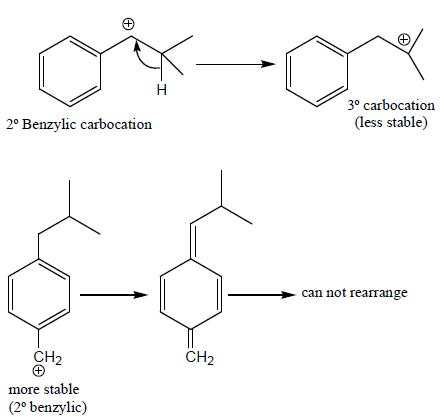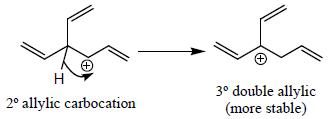*Answer can only contain numeric values
QUESTION: 19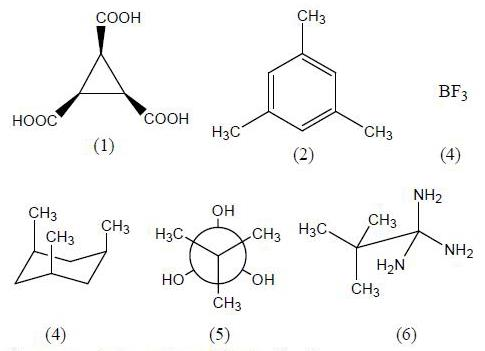How many above compounds having C3 axis.

Solution:

All six structures have C3 axis.

*Answer can only contain numeric values
QUESTION: 20

How many compounds (given below) shows three signals in new 13C-NMR spectrum ______ O-xylene, p-xylene, 1, 4-dimethoxybenzene, trans-1,2-dimethylcyclopropane, 4-bromoanisole, cis-1, 2 dimethylcyclopropane, Resorcinol

Solution: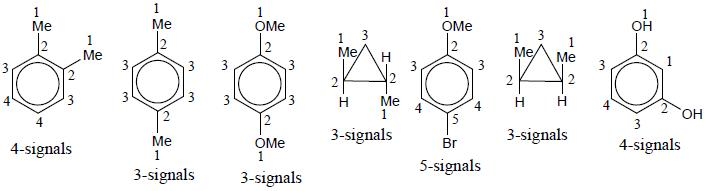*Answer can only contain numeric values
QUESTION: 21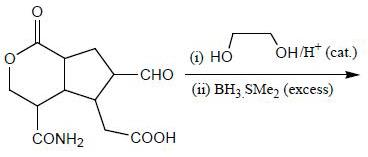How many functional groups will involve in reduction process?

Solution: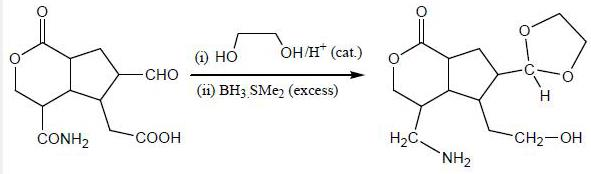Total number of functional groups reduced are two